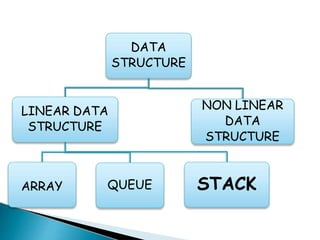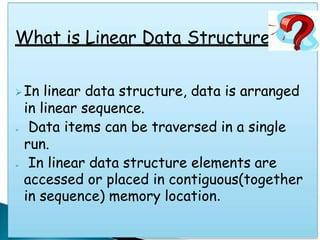Successfully reported this slideshow.

# Stack_Data_Structure.pptx×

1 of 19
1 of 19

# Stack_Data_Structure.pptx

Stack Data Structure
Stack
LIFO
Last in First Out
Stack in data Structure

Stack Data Structure
Stack
LIFO
Last in First Out
Stack in data Structure

## More Related Content

### Stack_Data_Structure.pptx

1. 1. STACK
2. 2. DATA STRUCTURE LINEAR DATA STRUCTURE ARRAY QUEUE STACK NON LINEAR DATA STRUCTURE
3. 3. What is Linear Data Structure In linear data structure, data is arranged in linear sequence.  Data items can be traversed in a single run.  In linear data structure elements are accessed or placed in contiguous(together in sequence) memory location.
4. 4. WHAT Is  A stack is called a last-in-first-out (LIFO) collection. This means that the last thing we added (pushed) is the first thing that gets pulled (popped) off. A stack is a sequence of items that are accessible at only one end of the sequence.
5. 5. Definition of Stack: Stacks in Data Structures is a linear type of data structure that follows the LIFO (Last-In-First-Out) principle and allows insertion and deletion operations from one end of the stack data structure, that is top. If any Element inserted then Top will be increased by 1 & if any Element deleted then Top will be decreased by 1. Top=Top+1 (Insertion) Top=Top-1 (Deletion)
6. 6. EXAMPLES OF STACK:
7. 7. Operations that can be performed on STACK:  PUSH.  POP.
8. 8. PUSH : It is used to insert items into the stack. POP: It is used to delete items from stack. TOP: It represents the current location of data in stack.
9. 9. ALGORITHM OF INSERTION IN STACK: (PUSH) 1. Insertion(a,top,item,max) 2. If top=max then print ‘STACK OVERFLOW’ exit else 3. top=top+1 end if 4. a[top]=item 5. Exit
10. 10. ALGORITHM OF DELETION IN STACK: (POP) 1. Deletion(a,top,item) 2. If top=0 then print ‘STACK UNDERFLOW’ exit else 3. item=a[top] end if 4 . top=top-1 5. Exit
11. 11. ALGORITHM OF DISPLAY IN STACK: 1.Display(top,i,a[i]) 2.If top=0 then Print ‘STACK EMPTY’ Exit Else 3.For i=top to 0 Print a[i] End for 4.exit
12. 12. APPLICATIONS OF STACKS ARE: I. Reversing Strings: • A simple application of stack is reversing strings. To reverse a string , the characters of string are pushed onto the stack one by one as the string is read from left to right. • Once all the characters of string are pushed onto stack, they are popped one by one. Since the character last pushed in comes out first, subsequent pop operation results in the reversal of the string.
13. 13. For example: To reverse the string ‘REVERSE’ the string is read from left to right and its characters are pushed . LIKE: onto a stack.
14. 14. II. Checking the validity of an expression containing nested parenthesis: • Stacks are also used to check whether a given arithmetic expressions containing nested parenthesis is properly parenthesized. • The program for checking the validity of an expression verifies that for each left parenthesis braces or bracket ,there is a corresponding closing symbol and symbols are appropriately nested.
15. 15. For example: VALID INPUTS INVALID INPUTS { } { ( } ( { [ ] } ) ( [ ( ( ) ] ) { [ ] ( ) } { } [ ] ) [ { ( { } [ ] ( { [ { ) } ( ] } ] })}]
16. 16. III. Evaluating arithmetic expressions: INFIX notation: The general way of writing arithmetic expressions is known as infix notation. e.g, (a+b) PREFIX notation: e.g, +AB POSTFIX notation: e.g: AB+
17. 17. Conversion of INFIX to POSTFIX conversion: Example: 2+(4-1)*3 2+41-*3 2+41-3* 241-3*+ step1 step2 step3 step4
18. 18. CURREN T SYMBOL ACTION PERFORME D STACK STATUS POSTFIX EXPRESSIO N ( PUSH C C 2 2 2 + PUSH + (+ 2 ( PUSH ( (+( 24 4 24 - PUSH - (+(- 241 1 POP 241- ) (+ 241- * PUSH * (+* 241- 3 241-3 POP * 241-3* POP + 241-3*+ CONVERSION OF INFIX INTO POSTFIX 2+(4-1)*3 into 241-3*+
19. 19. THANK YOU